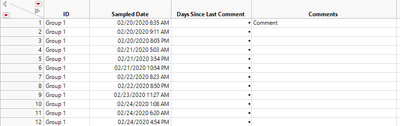Our World Statistics Day conversations have been a great reminder of how much statistics can inform our lives. Do you have an example of how statistics has made a difference in your life? Share your story with the Community!
Choose Language Hide Translation Bar
Highlighted

## Date Difference calculation referencing grouping columns

I am trying to calculate a Date Difference in Days between the current row and the last row where a comment is non-missing. Any help greatly appreciated!

``````If( (:ID == Lag( :ID ) | Row() == 1) & !Is Missing( :Comments ), 0,
Date Difference( :Sampled Date, Lag(:Sampled Date, n=last row where !Is Missing(:Comments)), "Day" )
)``````1 ACCEPTED SOLUTION

Accepted Solutions
Highlighted

## Re: Date Difference calculation referencing grouping columns

Here is the formula that I would use for the calculation

``````If( Row() == 1 | :ID != Lag( :ID ),
lastCmtDate = .
);
lastCmtDate = :Sampled Date
);
:Days Since Last Comment = (:Sampled Date - lastCmtDate) / In Days( 1 );``````
Jim
4 REPLIES 4
Highlighted

## Re: Date Difference calculation referencing grouping columns

If the table is always sorted by date you could do this with two columns, as shown below.

``````New Column( "Last Comment Date",
Numeric,
"Continuous",
Format( "m/d/y h:m", 19 ),
Input Format( "Monddyyyy h:m" ),
Formula(
If(
Row() == 1, :Sampled Date,
:Sampled Date
)
),
Set Display Width( 136 )
);
New Column( "Days Since Last Comment New",
Numeric,
"Continuous",
Format( "Best", 12 ),
Formula( (:Sampled Date - :Last Comment Date) / (3600 * 24) ),
Set Display Width( 109 )
);``````
Highlighted

## Re: Date Difference calculation referencing grouping columns

Thanks ih, but this doesn't work. The new column you created is not populated with the date of the last comment, just the lag sampled date. Thanks for trying!

Highlighted

## Re: Date Difference calculation referencing grouping columns

Here is the formula that I would use for the calculation

``````If( Row() == 1 | :ID != Lag( :ID ),
lastCmtDate = .
);